## Find the third term of a geometric progression if the sum of the first three terms is equal to 12, and the sum of the first six terms is equ

Question

Find the third term of a geometric progression if the sum of the first three terms is equal to 12, and the sum of the first six terms is equal to (−84).

in progress 0
2 months 2021-07-24T22:48:06+00:00 1 Answers 4 views 0

1. Given:

The sum of the first three terms = 12

The sum of the first six terms = (−84).

To find:

The third term of a geometric progression.

Solution:

The sum of first n term of a geometric progression is: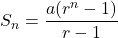Where, a is the first term and r is the common ratio.

The sum of the first three terms is equal to 12, and the sum of the first six terms is equal to (−84).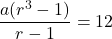…(i)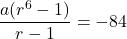…(ii)

Divide (ii) by (i), we get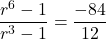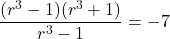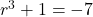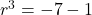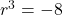Taking cube root on both sides, we get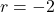Puttingin (i), we get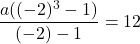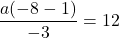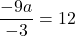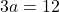Divide both sides by 3.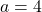The nth term of a geometric progression is: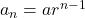Where, a is the first term and r is the common ratio.

Putting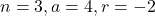in the above formula, we get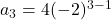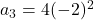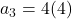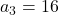Therefore, the third term of the geometric progression is 16.